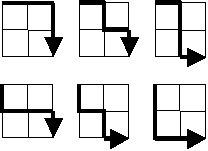# A Square Grid Path Problem

Last November I solved Problem 15 of Project Euler (a counting problem involving paths in square grids), and, although the problem admits a simple solution, some of the solutions presented in their forums are very complicated. Thus, I thought it would be a good idea to present my solution, as I consider it very simple.

## Problem statement

Starting in the top left corner of a $2{\times}2$ grid, there are 6 routes (without backtracking) to the bottom right corner.How many routes are there through a 20×20 grid?

## My solution

In order to make the problem more interesting, let us investigate the more general problem of counting the number of routes in an $n{\times}n$ grid. Our argument is based on three observations:

1. all the paths have size $2{\times}n$ (the reason is obvious: you have to go right $n$ positions and down another $n$ positions);

2. since we can only go right or down, we can identify every path by a string of Rs and Ds, where an R means going right and a D means going down; as an example, the paths illustrated in the problem statement are (from left to right and from top to bottom): RRDD, RDRD, RDDR, DRRD, DRDR and DDRR;

3. the strings mentioned above must contain the same number of Rs and Ds.

From these three observations, we can transform the problem to the following:

How many different strings of size $2{\times}n$, consisting of n Rs and n Ds, are there?

The solution is now very simple, because the positioning of $n$ Ds (or Rs) determines the positioning of the other $n$ Rs (or Ds). Hence, the number we are interested in is the number of ways in which we can choose $n$ positions from $2{\times}n$ available positions. The answer, using the traditional notation for the binomial coefficient, is:

$${2n \choose n} = \frac{(2n)!}{n! \times n!}~~~~.$$

Instantiating $n$ with 20, we get the answer to the initial problem of the $20{\times}20$ grid.

## Generalization to $m{\times}n$ grids

The generalization to an $m{\times}n$ grid is also simple. The only difference is that the strings have length $m+n$. Using the same reasoning as above, the number of paths through an $m{\times}n$ grid is:

$${m+n \choose n} = \frac{(m+n)!}{m!\times n!}~~~~.$$

Final note: If you want to access the forum of the problem, you have to solve it.###### Computer Scientist

My research interests include software reliability, software verification, and formal methods applied to software engineering. I am also interested in interactive storytelling. For more details, see some of my projects or my selected (or recent) publications. More posts are available in my blog. Follow me on Twitter or add me on LinkedIn.